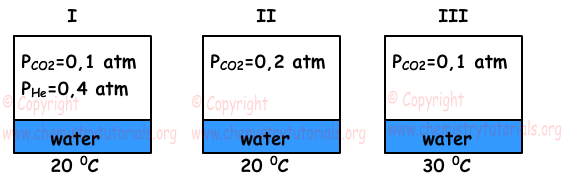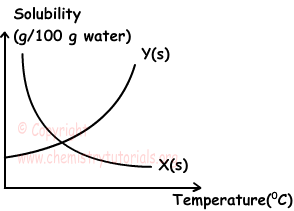## Solutions Exam1 and Problem Solutions

Solutions Exam1 and  Problem Solutions

1. X solid has ionic structure and solubility of it increases with increasing temperature. If solubility of X in water at 15 0C is 20g X/100 g water, find which ones of the following statements are true for solution prepared by using 10 g X and 50 g water at 15 0C;

I. This solution conducts electric current

II. If 30 g water and 5 g X are added to solution under constant temperature, some amount of X stays undissolved.

III. If solution is cooled from 15 0C to 10 0C, some amount of  crystallize.

Solution:

I. Since structure of X is ionic, when it dissolves in water it decompose into its ions and we know that solutions including ions conduct electric current. I is true.

II. After addition of water and X we have;

Mass of water=50+30=80 g

Mass of X=10+5=15 g

At 15 0C;

100g water dissolves 20 g X

80g water dissolves ? g X

¯¯¯¯¯¯¯¯¯¯¯¯¯¯¯¯¯¯¯¯¯¯¯¯¯¯

?=16 g X can be dissolved in 80 g water. Since we have 15 X, solution is unsaturated, so all X is dissolved. II is false.

III. At 15 0C;

100 g water dissolves 20 g X

50 g water dissolves ? g X

¯¯¯¯¯¯¯¯¯¯¯¯¯¯¯¯¯¯¯¯¯¯¯¯¯¯

?=10 g X can be dissolved.

This solution is saturated. Thus, since it is endothermic solution ( solubility increases with increasing temperature), when we cool it some X crystallize. III is true.

2. There are equal amounts of water in given containers.Find relation between solubility of CO2 gas in water under given conditions.

Solution:

Solubility of CO2 in water increases with increasing partial pressure of CO2 and decreasing in the temperature. I and II has equal temperature but partial pressure of CO2 in II is larger than I. So, solubility of II is larger than I.

I and III has equal partial pressure, but temperature of I is lower than III, so solubility of I is larger than III.Relation between them become;

II > I > III

3. Graph given below shows relation between solubility and temperature of X and Y solids.Which one of the following statements are false for this graph?

I. Dissolution of X in water is exothermic

II. When X solid and X-water solution in equilibrium is heated, amount of solid X decreases

III. When saturated Y solution is heated, it becomes unsaturated.

Solution:

I. Since solubility of X decreases with increasing temperature, it is exothermic. I is true.

II. Since solution of X in water is exothermic, when solution is heated, some amount of X crystallize so amount of X solid increases. II is false.

III. As you can see from the graph, solubility of Y in water is endothermic. Increasing temperature increases its solubility and saturated solution becomes unsaturated. III is true.

4. 25 g salt and 125 g water are mixed and solution is prepared. Find concentration of solution by percent mass.

Solution:

Mass of Solute: 25 g

Mass of Solution: 25 + 125 = 150 g

125 g solution includes 25 g solute

100 g solution includes X g solute

¯¯¯¯¯¯¯¯¯¯¯¯¯¯¯¯¯¯¯¯¯¯¯¯¯¯¯

X=20 g %

Or using formula;

Percent by mass=25.100/125=20 %

5. How much water must be vaporized from 0,4 molar 200 mL H2SO4 solution to make it 1,6 molar at same temperature?

Solution:

V=200 mL = 0,2 L

Mole of H2SO4;

n=0,4.0,2=0,08mol

n=M.V, M=n/V

1,6 molar=0,08/V=0,05L=50mL

200-50=150mL water must be vaporized.

Related